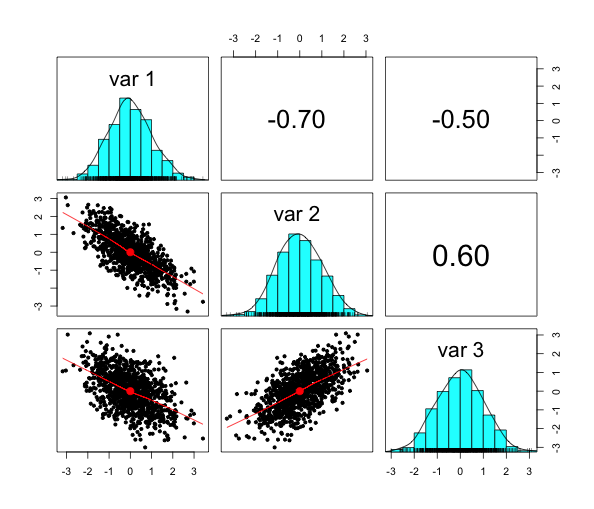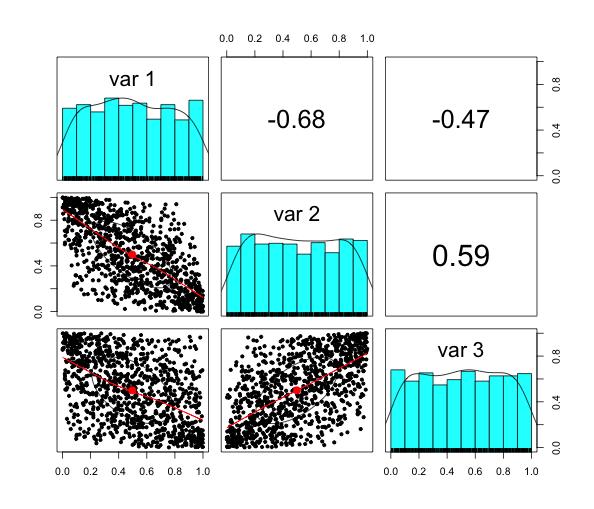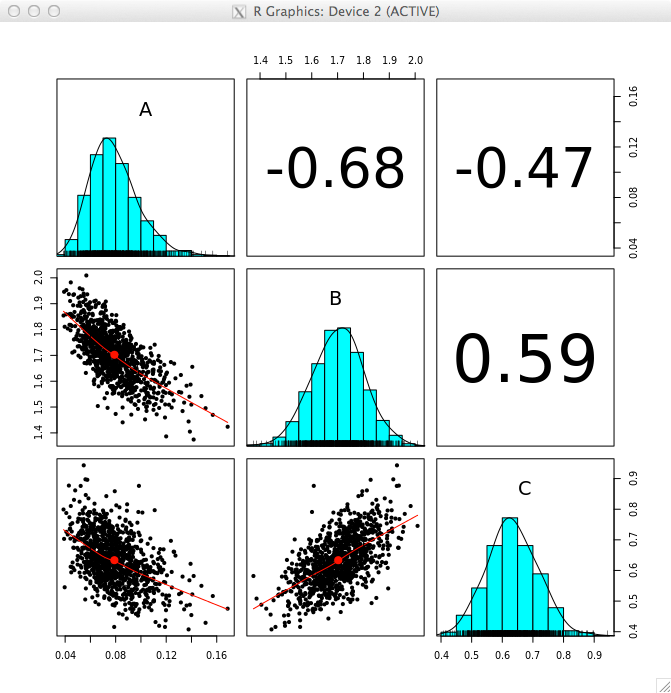# Generating and visualising multivariate random numbers in R

This post will present the wonderful pairs.panels function of the psych package  that I discovered recently to visualise multivariate random numbers.

Here is a little example with a Gaussian copula and normal and log-normal marginal distributions. I use pairs.panels to illustrate the steps along the way.

library(psych)
library(MASS)
Sig <- matrix(c(1, -0.7, -.5,
-0.7, 1, 0.6,
-0.5, 0.6, 1),
nrow=3)
X <- mvrnorm(1000, mu=rep(0,3), Sigma = Sig,
empirical = TRUE)
pairs.panels(X)Next, I map the random figures into the interval [0,1] using the distribution function pnorm, so I end up with multivariate uniform random numbers:

U <- pnorm(X)
pairs.panels(U)Finally, I transform the uniform numbers into the desired marginals:

Z <- cbind(
A=qlnorm(U[,1], meanlog=-2.5, sdlog=0.25),
B=qnorm(U[,2], mean=1.70, sd=0.1),
C=qnorm(U[,3], mean=0.63,sd=0.08)
)
pairs.panels(Z)Those steps can actually be shorten with functions of the copula package .

Unfortunately, I struggled to install the copula package on my local Mac, running Mavericks. No CRAN binaries are currently available and gfortran is playing up on my system to install it from source. Thus, I quickly fired up an Ubuntu virtual machine on Amazon’s EC2 Cloud and installed R. Within 15 minutes I was back in business - that is actually pretty amazing.

library(copula)
myCop=normalCopula(param=c(-0.7,-.5,0.6), dim = 3, dispstr = "un")
myMvd <- mvdc(copula=myCop, margins=c("lnorm", "norm", "norm"),
paramMargins=list(list(meanlog=-2.5, sdlog=0.25),
list(mean=1.70, sd=0.1),
list(mean=0.63,sd=0.08)) )
Z2 <- rmvdc(myMvd, 1000)
colnames(Z2) <- c("A", "B", "C")
pairs.panels(Z2)### References

 Revelle, W. (2014) psych: Procedures for Personality and Psychological Research, Northwestern University, Evanston, Illinois, USA, http://CRAN.R-project.org/package=psych Version = 1.4.5.

 Marius Hofert, Ivan Kojadinovic, Martin Maechler and Jun Yan (2014). copula: Multivariate Dependence with Copulas. http://CRAN.R-project.org/package=copula Version = 0.999-10

### Session Info Local

R version 3.1.0 (2014-04-10)
Platform: x86_64-apple-darwin13.1.0 (64-bit)

locale:
 en_GB.UTF-8/en_GB.UTF-8/en_GB.UTF-8/C/en_GB.UTF-8/en_GB.UTF-8

attached base packages:
 stats graphics  grDevices utils datasets methods base

other attached packages:
 psych_1.4.5 MASS_7.3-31

loaded via a namespace (and not attached):
 tools_3.1.0

### Session Info EC2

R version 3.0.2 (2013-09-25)
Platform: x86_64-pc-linux-gnu (64-bit)

locale:
 LC_CTYPE=en_US.UTF-8       LC_NUMERIC=C
 LC_TIME=en_US.UTF-8        LC_COLLATE=en_US.UTF-8
 LC_MONETARY=en_US.UTF-8    LC_MESSAGES=en_US.UTF-8
 LC_PAPER=en_US.UTF-8       LC_NAME=C
 LC_MEASUREMENT=en_US.UTF-8 LC_IDENTIFICATION=C

attached base packages:
 stats graphics  grDevices utils datasets  methods   base

other attached packages:
 psych_1.4.5 copula_0.999-10

loaded via a namespace (and not attached):
 stats4_3.0.2